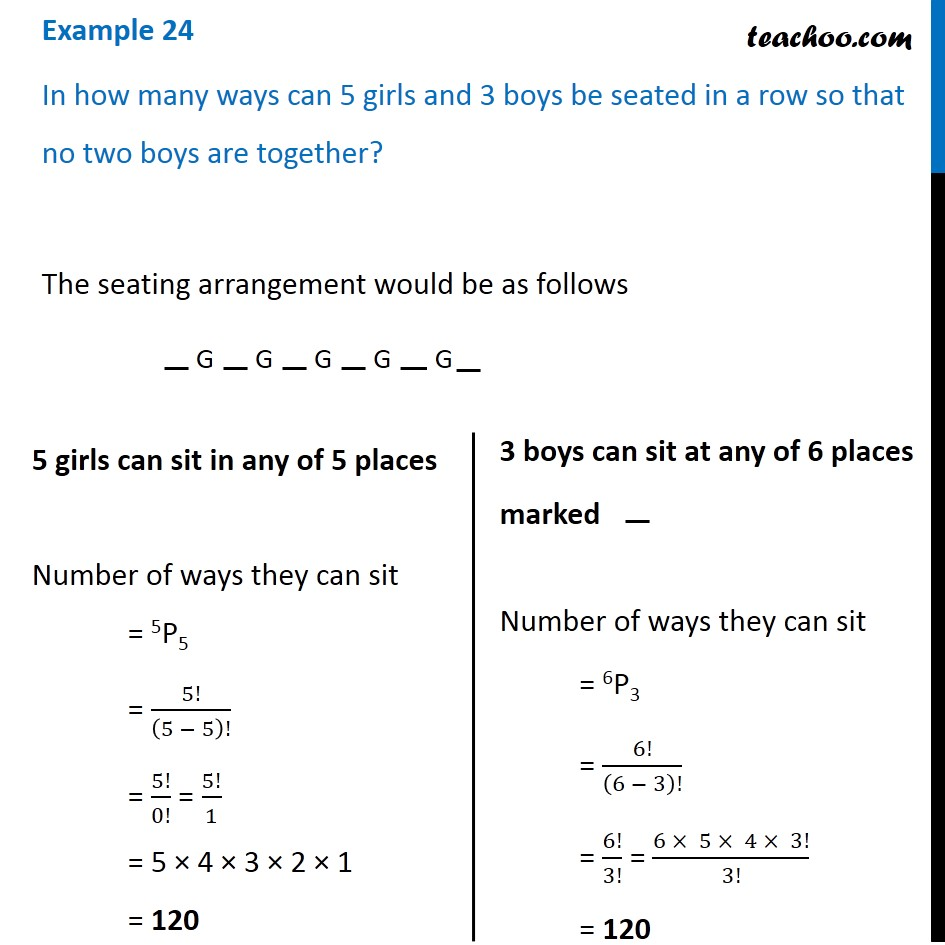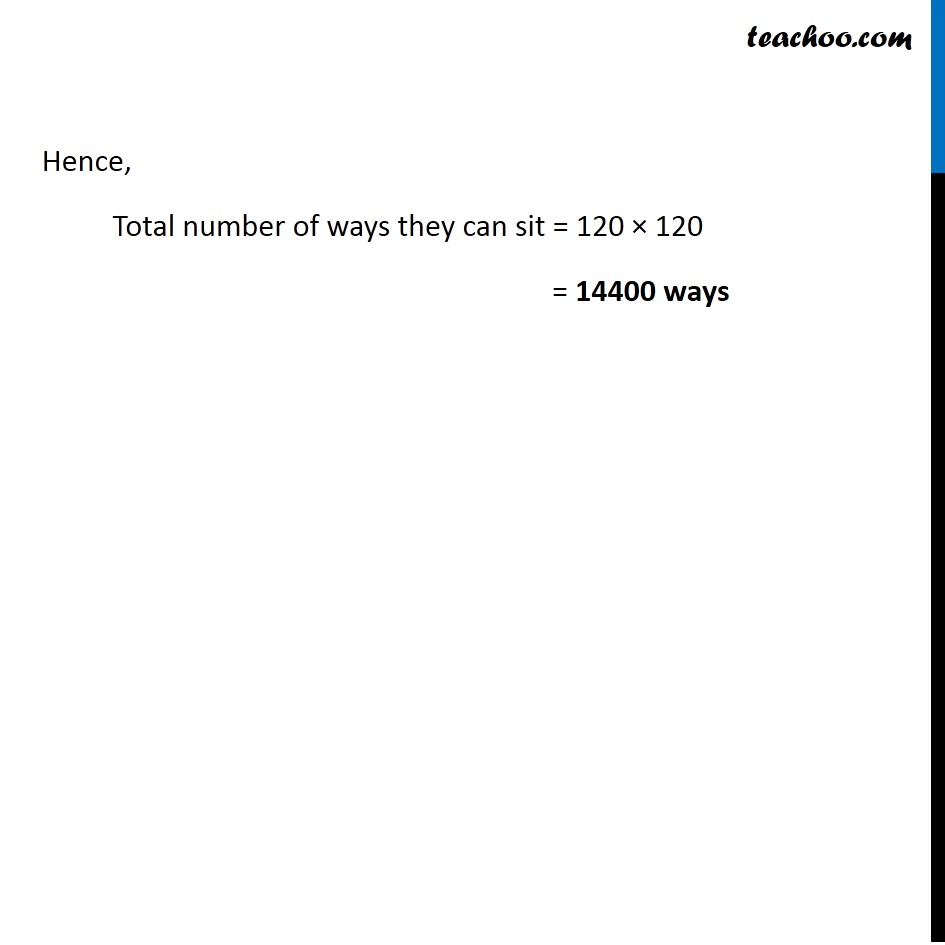Examples

Chapter 6 Class 11 Permutations and Combinations
Serial order wiseLearn in your speed, with individual attention - Teachoo Maths 1-on-1 Class

### Transcript

Example 24 In how many ways can 5 girls and 3 boys be seated in a row so that no two boys are together? The seating arrangement would be as follows Hence total number of ways they can sit = 120 × 120 = 14400 ways 5 girls can sit in any of 5 places Number of ways they can sit = 5P5 = 5!/(5 − 5)! = 5!/0! = 5!/1 = 5 × 4 × 3 × 2 × 1 = 120 3 boys can sit at any of 6 places marked Number of ways they can sit = 6P3 = 6!/(6 − 3)! = 6!/3! = (6 × 5 × 4 × 3!)/3! = 120